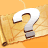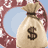# What is the Impact of Numerology on Your LifeNumerology is an important aspect of astrology, which helps in determining the life and future of a person through numbers. Numerology is used to determine the thought process, life, future, career path etc. of an individual through the medium of numbers and mathematical calculations. In simple terms, it deals with the predictions in relation to numbers. It is also known as Anka Vidya, Sankhya Shastra, Anka Shastra etc. Numerological calculations are based upon the characteristics of nine planets, i.e, Jupiter, Mercury, Mars, Uranus, Saturn, Venus, Varun including Moon and Sun. The roots of numerology can be traced back some 10,000 years to Egypt, Greece, Babylonia and with the contribution of Pythagoras, it got its significant place in the world. Numbers are a part of each day that rises and sets. Pythagoras explaining the importance of numbers, said, “Number rules the universe’’ . Statistics is another science for studying the numbers. It comprises of facts, figures, calculation of incidents etc. But Statistics is totally different from Numerology. In ancient times, the knowledge of Numerology was confined to Indians, Greeks, Egyptians and Chinese people. In India, numbers are very well used in other astrology forms like prashna kundali (question based horoscope), swarodam shastra etc. It is very difficult to define Numerology in one line. As per the Numerologists, it is believed that Numerology first used Hebrew system of numbers. As a subject, it belongs to Hebrews and along with them, Egyptians and Gypsies played an important role in the foundation and development of Numerology. Hebrew system of Numerology contains 22 numbers and each letter has been assigned one number ranging from 1 to 22. Hebrew uses numbers in place of letters and vice versa. The numbers and letters are governed by the position of different planets and zodiac signs. Thus, it can be said that the relation between numbers, letters, planets and signs was established during this period. In Numerology, each planet has been assigned a number ranging from1 to 9 depending on the effect of number on a particular planet. Thus, these nine planets play a significant role in the life of an individual. The position of planets at the time of birth give an insight about specific characteristics or personality traits of an individual. If the number of one person gets along with the number of other, they share a healthy relationship. Numerology studies the name of an individual to get a specified number. Each letter is assigned a number and summation of these letters gives a number on the basis of which the future of a person can be determined. Along with it, the calculation of Destiny number is also important that is based on the date of birth.

## Ancient and Modern System of Numerology

Ancient and Modern Numerologists have adopted the following systems of Numerology In Western countries, Numerologists like Goodman, Montroj, Moris, James Lee, Helen Hitchcock etc. have adopted different systems of numerology. The system developed by Dr. Cross Montroj, Sephrial etc. is also knows as Hebrew Numerology or Old method. The numbers assigned to this system are in sequence of Hebrew alphabets. Whereas, as Modern Numerologists like James lee, Hitchcock, Taylor etc. have assigned the numbers to the alphabets that are used in modern times, their method is knows as Modern Numerology. Unlike Vedic astrology, Numerology does not involve difficult calculations. In case a person is not aware of his birth time, then date of birth and name can be used to determine the career path, life path and future events related to a person. Important information can be obtained by the means of Numerology. One can excel in Numerology with the knowledge of English and Numerology. Ancient scholars like Ciero, Montroj, Dr. Cross accepted the relation between the planets and numbers which are as follows : Sun  - Positive Points - 1 : Negative Points -  4 Moon -  Positive Points - 7  : Negative Points - 2 Mars : 9, Jupiter - 3, Mercury - 5, Venus - 6, Saturn - 8, Uranus - 4, Neptune - 7 Modern day Numerologists; Taylor, James lee, Helan Hichkok, Goodmen etc. do not accept this relation and have made some changes to it. Following is the list of numbers assigned to each planet : Sun - 1 , Moon - 2 , Jupiter - 3, Saturn - 4 , Mercury - 5 , Venus - 6, Uranus - 7, Mars - 8, Neptune - 9, Pluto - 0 Some modern Numerologists do their calculations without co-relating the numbers and the planets. Hebrew letters have been assigned following numbers. The sequence of these letters is different from the sequence of modern English alphabets. A, B, C, D, E, F, G, H, I, J, K, L, M 1, 2, 3, 4, 5, 8, 3, 5, 1, 1, 2, 3, 4, N, O, P, Q, R, S, T, U, V, W, X, Y, Z, 5, 7, 8, 1, 2, 3, 4, 6, 6, 6, 5, 1, 7 In the old system, number nine is not used in the calculation and does not even follow any specific sequence. But in modern system, number nine has been brought into use which is as follows : A, B, C, D, E, F, G, H, I, 1, 2, 3, 4, 5, 6, 7, 8, 9, J, K, L, M, N, O, P, Q, R, 1, 2, 3, 4, 5, 6, 7, 8, 9, S, T, U, V, W, X, Y, Z, 1, 2, 3, 4, 5, 6, 7, 8, Numbers such as  11, 12 and 33 have been assigned a distinctive place in the modern system of Numerology, but not in the old system. Numbers in the range of 0-9 are major numbers. Some scholars do not use 0 in their calculations, but do accept its importance. In addition to nine points, numbers ahead of 10 are called mixed. Numbers are of two types. The first type ranging from 0 to 9 are the major numbers and numbers exceeding 10 are mixed numbers. To get a single digit number, the mixed numbers are converted into major numbers. For example, 22 = 2+2 = 4. Florence Campbell states, “People who possess Master numbers (11 and 22) are born leaders”.

## Root Number

Root number is based on the date of your birth, i.e, the date on which a person is born. If a person was born on 2nd January, then the root number will be 2.

## Destiny number

It is calculated on the basis of date of birth which includes the month, year and date. For Example : For instance, a person is born on 14th December 1942, then the procedure to calculate the Destiny number is as follows : Date of birth + Month of birth + Year of Birth =  Destiny number Date of birth - 1+ 4 = 5 Month of Birth - 1+ 2 = 1 + 2 = 3 Year of Birth - 1942 = 1+ 9 + 4 + 2 = 16 = 1 + 6 = 7 The destiny number of this person will be 6 ( 5 + 3 + 7 = 15 = 6 )

## Name Number

We can calculate the number of any name and place with the help of the number table. For example, if we have to take out the name number of Anil Kumar, then the method to calculate the number will be as follows: A N I L        K U M A R 1 5 1 3        2 6 4 1 2 1+5+1+3=10,  2+6+4+1+2=15 10+15=25 2+5=7 The name number of this person is 7.

## Numbers Assigned to Each Zodiac Sign

Aries - 1, Taurus - 2, Gemini - 3, Cancer - 4, Leo - 5, Virgo - 6, Libra - 7, Scorpio - 8, Sagittarius - 9 Two digit numbers beyond 1-9 such as 10, 11 etc. are again summed up and brought into a single digit. Capricorn - 10  = 1+0 = 1,  Aquarius - 11 = 1+1 = 2, Pisces - 12 = 1+2 =3 To get your Numerology Life Analysis Report, please click on this link : Numerology Life Analysis
Categories: